# ICSE Class 8 Maths Selina Solutions for Chapter 15 Linear Inequations

ICSE Class 8 Maths Selina Solutions are useful for students to score well in their examination. For the benefit of the students, we have provided detailed solutions of the Chapter 15 Linear Inequations. The solutions contain elaborate steps covering all the problems that are mentioned under the exercise. The Selina Solutions have been created by the experts by explaining each and every step. This will also help students in fetching more marks in the annual exam if they write the answers as provided in the Selina solution pdf.

Solving these questions mainly help students to clear all the concepts about Linear Inequations. Students can download ICSE Class 8 Maths Selina Solutions Chapter 15 Linear Inequations in pdf format from the link provided  below. They can also use this pdf for reference during revision. Click on the link below to download the ICSE Class 8 Maths Selina solutions of Linear Inequations.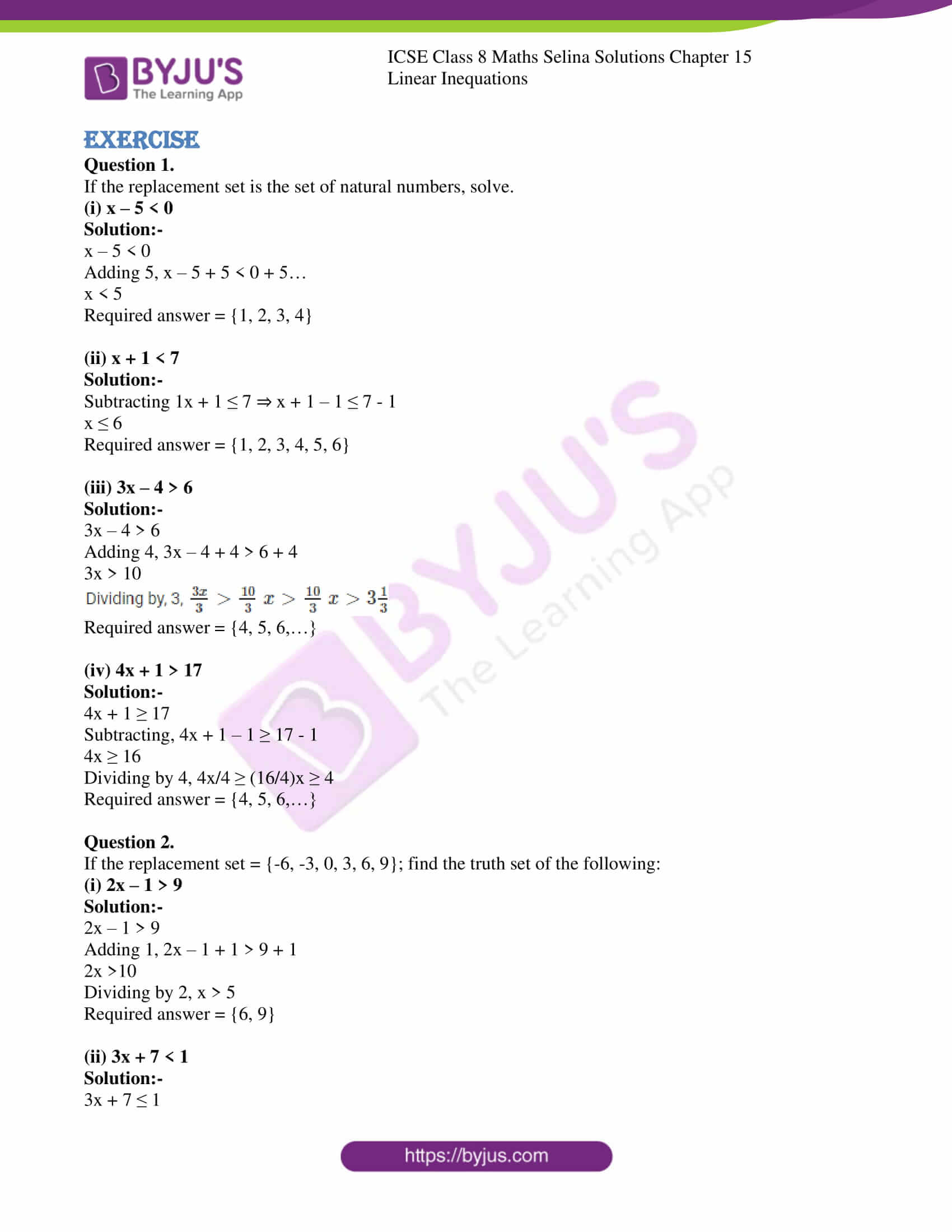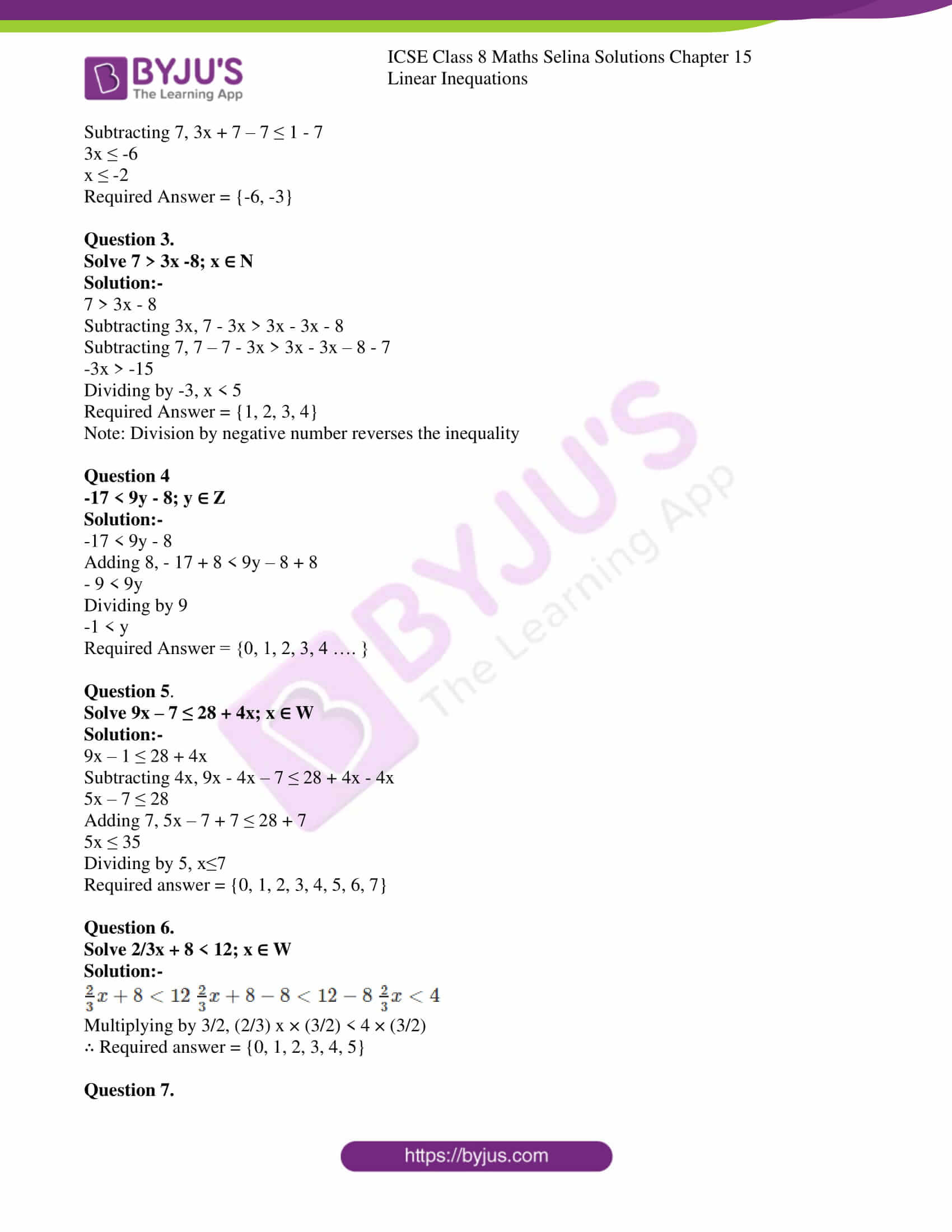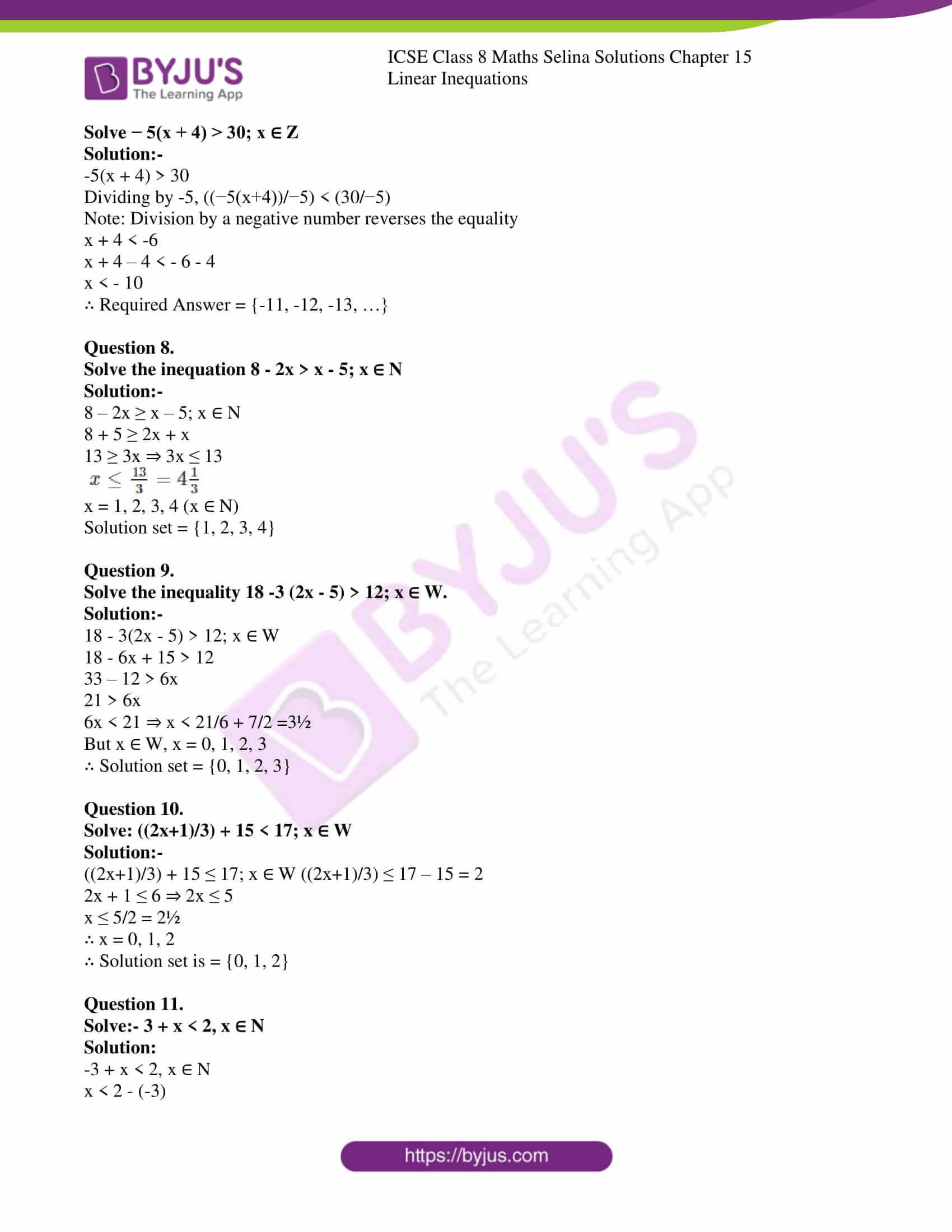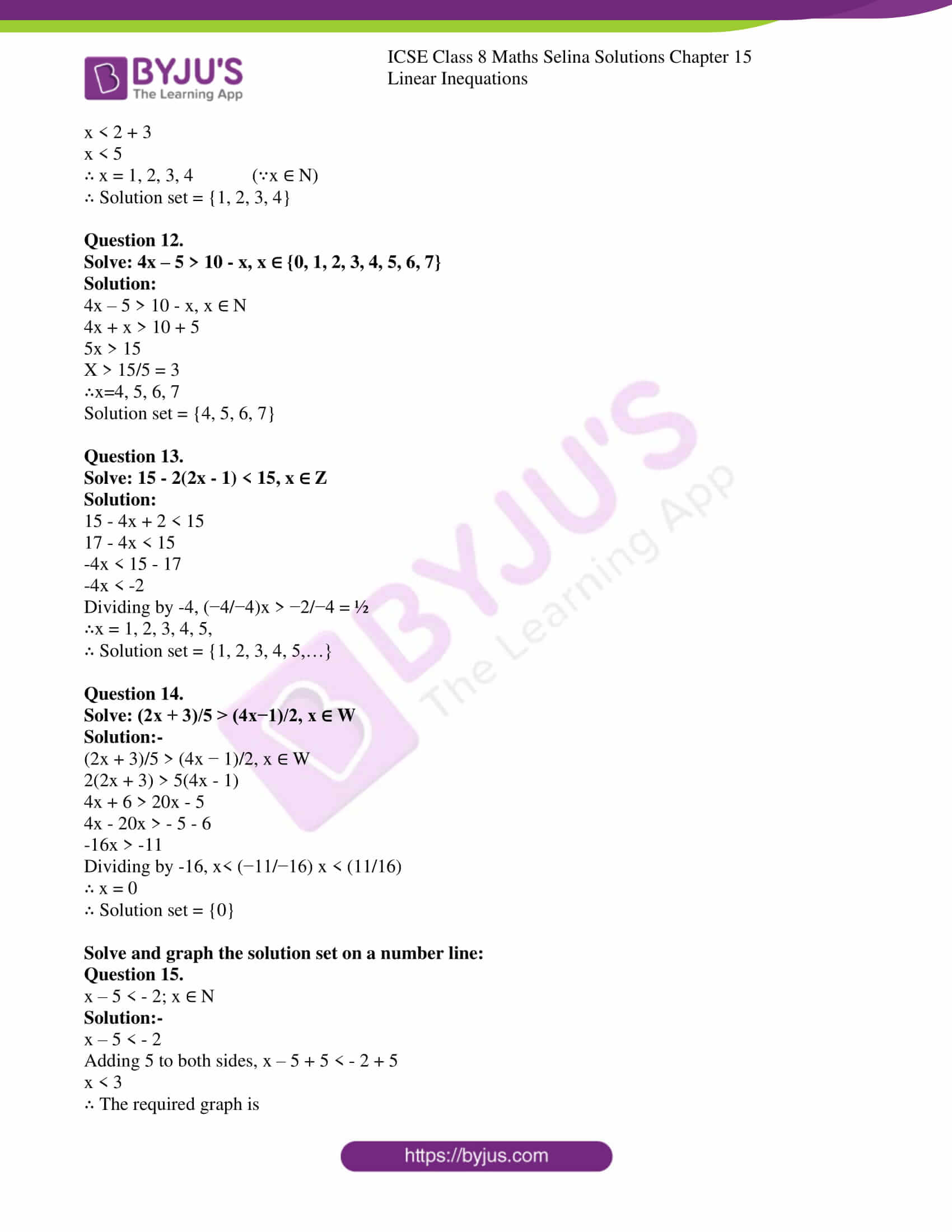Chapter 15 of ICSE Class 8 Maths Selina Solutions deals with the Linear Inequations. It has one exercise that contains a total of 15 questions. The detailed step by step solutions of the questions has been mentioned below.

### CHAPTER 15 – LINEAR INEQUATIONS

Exercise

Question 1.

If the replacement set is the set of natural numbers, solve.

(i) x – 5 < 0

Solution:-

x – 5 < 0

Adding 5, x – 5 + 5 < 0 + 5…

x < 5

Required answer = {1, 2, 3, 4}

(ii) x + 1 < 7

Solution:-

Subtracting 1x + 1 ≤ 7 ⇒ x + 1 – 1 ≤ 7 – 1

x ≤ 6

Required answer = {1, 2, 3, 4, 5, 6}

(iii) 3x – 4 > 6

Solution:-

3x – 4 > 6

Adding 4, 3x – 4 + 4 > 6 + 4

3x > 10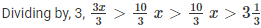Required answer = {4, 5, 6,…}

(iv) 4x + 1 > 17

Solution:-

4x + 1 ≥ 17

Subtracting, 4x + 1 – 1 ≥ 17 – 1

4x ≥ 16

Dividing by 4, 4x/4 ≥ (16/4)x ≥ 4

Required answer = {4, 5, 6,…}

Question 2.

If the replacement set = {-6, -3, 0, 3, 6, 9}; find the truth set of the following:

(i) 2x – 1 > 9

Solution:-

2x – 1 > 9

Adding 1, 2x – 1 + 1 > 9 + 1

2x >10

Dividing by 2, x > 5

(ii) 3x + 7 < 1

Solution:-

3x + 7 ≤ 1

Subtracting 7, 3x + 7 – 7 ≤ 1 – 7

3x ≤ -6

x ≤ -2

Question 3.

Solve 7 > 3x -8; x ∈ N

Solution:-

7 > 3x – 8

Subtracting 3x, 7 – 3x > 3x – 3x – 8

Subtracting 7, 7 – 7 – 3x > 3x – 3x – 8 – 7

-3x > -15

Dividing by -3, x < 5

Required Answer = {1, 2, 3, 4}

Note: Division by negative number reverses the inequality

Question 4

-17 < 9y – 8; y ∈ Z

Solution:-

-17 < 9y – 8

Adding 8, – 17 + 8 < 9y – 8 + 8

– 9 < 9y

Dividing by 9

-1 < y

Required Answer = {0, 1, 2, 3, 4 …. }

Question 5.

Solve 9x – 7 ≤ 28 + 4x; x ∈ W

Solution:-

9x – 1 ≤ 28 + 4x

Subtracting 4x, 9x – 4x – 7 ≤ 28 + 4x – 4x

5x – 7 ≤ 28

Adding 7, 5x – 7 + 7 ≤ 28 + 7

5x ≤ 35

Dividing by 5, x≤7

Required answer = {0, 1, 2, 3, 4, 5, 6, 7}

Question 6.

Solve 2/3x + 8 < 12; x ∈ W

Solution:-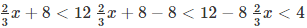Multiplying by 3/2, (2/3) x × (3/2) < 4 × (3/2)

∴ Required answer = {0, 1, 2, 3, 4, 5}

Question 7.

Solve − 5(x + 4) > 30; x ∈ Z

Solution:-

-5(x + 4) > 30

Dividing by -5, ((−5(x+4))/−5) < (30/−5)

Note: Division by a negative number reverses the equality

x + 4 < -6

x + 4 – 4 < – 6 – 4

x < – 10

∴ Required Answer = {-11, -12, -13, …}

Question 8.

Solve the inequation 8 – 2x > x – 5; x ∈ N

Solution:-

8 – 2x ≥ x – 5; x ∈ N

8 + 5 ≥ 2x + x

13 ≥ 3x ⇒ 3x ≤ 13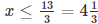x = 1, 2, 3, 4 (x ∈ N)

Solution set = {1, 2, 3, 4}

Question 9.

Solve the inequality 18 -3 (2x – 5) > 12; x ∈ W.

Solution:-

18 – 3(2x – 5) > 12; x ∈ W

18 – 6x + 15 > 12

33 – 12 > 6x

21 > 6x

6x < 21 ⇒ x < 21/6 + 7/2 =3½

But x ∈ W, x = 0, 1, 2, 3

∴ Solution set = {0, 1, 2, 3}

Question 10.

Solve: ((2x+1)/3) + 15 < 17; x ∈ W

Solution:-

((2x+1)/3) + 15 ≤ 17; x ∈ W ((2x+1)/3) ≤ 17 – 15 = 2

2x + 1 ≤ 6 ⇒ 2x ≤ 5

x ≤ 5/2 = 2½

∴ x = 0, 1, 2

∴ Solution set is = {0, 1, 2}

Question 11.

Solve:- 3 + x < 2, x ∈ N

Solution:

-3 + x < 2, x ∈ N

x < 2 – (-3)

x < 2 + 3

x < 5

∴ x = 1, 2, 3, 4 (∵x ∈ N)

∴ Solution set = {1, 2, 3, 4}

Question 12.

Solve: 4x – 5 > 10 – x, x ∈ {0, 1, 2, 3, 4, 5, 6, 7}

Solution:

4x – 5 > 10 – x, x ∈ N

4x + x > 10 + 5

5x > 15

X > 15/5 = 3

∴x=4, 5, 6, 7

Solution set = {4, 5, 6, 7}

Question 13.

Solve: 15 – 2(2x – 1) < 15, x ∈ Z

Solution:

15 – 4x + 2 < 15

17 – 4x < 15

-4x < 15 – 17

-4x < -2

Dividing by -4, (−4/−4)x > −2/−4 = ½

∴x = 1, 2, 3, 4, 5,

∴ Solution set = {1, 2, 3, 4, 5,…}

Question 14.

Solve: (2x + 3)/5 > (4x−1)/2, x ∈ W

Solution:-

(2x + 3)/5 > (4x − 1)/2, x ∈ W

2(2x + 3) > 5(4x – 1)

4x + 6 > 20x – 5

4x – 20x > – 5 – 6

-16x > -11

Dividing by -16, x< (−11/−16) x < (11/16)

∴ x = 0

∴ Solution set = {0}

Solve and graph the solution set on a number line:

Question 15.

x – 5 < – 2; x ∈ N

Solution:-

x – 5 < – 2

Adding 5 to both sides, x – 5 + 5 < – 2 + 5

x < 3

∴ The required graph is### ICSE Class 8 Maths Selina Solutions Chapter 15 – Linear Inequations

Almost everyday we come across mathematical inequalities, but you might not have noticed them because they are so familiar. Some of the examples of mathematical inequalities that we come across in our daily lives are speed limit in highways, minimum payments on credit card bills, etc. While considering these situations we use mathematical thinking such as speed limits on highway can be mathematically written as Legal speed on the highway ≤ 65 miles per hour. When two expressions are connected by “greater than” or “less than” sign, we get an inequality. Also, the linear inequation is similar to a linear equation, where the equal to sign is replaced by the inequality sign.

Class 8 students can access ICSE Selina Solution Class 8 for all the other subjects which are available in BYJU’S website in downloadable pdf format.

Learn Maths & Science in an interactive & fun-loving way with BYJU’S App/Tablet and subscribe to YouTube Channel.